## conference papersJOURNAL OFAPPLIEDCRYSTALLOGRAPHY
ISSN: 1600-5767
Volume 40| Part s1| April 2007| Pages s269-s273

## Small-angle X-ray and small-angle neutron scattering investigations of colloidal dispersions of magnetic nanoparticles and clay nanoplatelets

aComplex Fluids Group, Institute of Physics, University of Brasilia, Brazil, bComplex Fluids Group, Institute of Chemistry, University of Brasilia, Brazil, cDepartment of Physics, Norwegian University of Science and Technology, Hoegskoleringen 5, N-7491 Trondheim, Norway, and dPhysics Department, Institute for Energy Technology, Kjeller, Norway
*Correspondence e-mail: jon.fossum@ntnu.no

(Received 16 August 2006; accepted 24 February 2007; online 6 April 2007)

We investigated mixed colloidal dispersions of clay platelets and magnetic nanoparticles using small-angle X-ray and neutron scattering. Our results show that the contribution to the scattering is essentially due to the magnetic nanoparticles. The scattering intensities are proportional to the concentration of magnetic particles, indicating that from the scattering point of view the sample is a colloidal dispersion of non-interacting magnetic objects, although the laponite and magnetic particles clearly interact when the sample textures are observed in an optical microscope. The visually observed phase separation may be characterized as a liquid–gas transition.

### 1. Introduction

It is a challenge in colloidal science to predict and monitor the thermodynamic stability of dispersions as well as to associate theoretical models to experimental results. Several technological and industrial applications require a stable colloid, and this is directly related to the process of agglomeration and phase separation. Colloidal stability involves several kinds of particle interactions such as van der Waals attraction, electrostatic repulsion between electric double layers and, in the case of ferrofluids, magnetic dipolar interactions. Considering the solvent as a continuous medium, it is possible to make analogies between colloidal and atomic systems, because the interparticle interaction potential has the same functional behavior in both cases (Israelachvili, 1985). In this context, liquid phases, gas phases and solid phases can be observed in colloidal dispersions. However, in contrast to atomic systems, the interparticle interactions in a colloid can be controlled by certain experimental parameters such as the ionic strength, the temperature, the colloid pH and in particular for ferrofluids the applied magnetic field. We study here a composite potentially smart material made by associating two aqueous colloidal systems, namely a laponite clay solution and a ferrofluid, with the purpose of obtaining a magnetorheological product. For both of these aqueous colloidal systems the one-phase behavior is a function of several parameters and depends strongly on the electrostatic interactions (Israelachvili, 1985). The magnetic nanoparticles used here, i.e. the ferrofluid, have already been associated with other complex fluids such as liquid crystals (Matuo et al., 2002). The chemical synthesis (Tourinho et al., 1990) and the colloidal stability (Campos et al., 2001) of such magnetic nanoparticles in aqueous media are relatively well known and their phase behavior as a function of the ionic strength and temperature has been discussed using small-angle neutron scattering (Dubois et al., 2000). Laponite (Fossum, 2000; Gabriel et al., 1996) is a widely studied synthetic clay that belongs to the family of swelling 2:1 clays (van Olphen, 1991). The colloidal phase diagram of aqueous laponite suspensions as a function of the ionic strength and the concentration of laponite has been discussed and includes four different regions (phases): isotropic liquid, isotropic gel, nematic gel and flocculation (Mourchid et al., 1998). Recently, maghemite magnetic nanoparticles were incorporated as a probe into clay dispersions in order to characterize their spatial repartition and microrheological behavior (Cousin et al., 2002). In the present work, we investigate the colloidal dispersion of clay platelets and magnetic nanoparticles using small-angle scattering experiments. A laponite clay dispersion (0.1 wt%) is synthesized in the isotropic liquid phase and mixed with a citrated ferrofluid based on cobalt ferrite nanoparticles in order to obtain a composite product. The parent laponite and ferrofluid colloids as well as the mixed composites are investigated by small-angle scattering experiments, which allow us to characterize the scattering objects in the composite samples as well as the colloidal stability of such laponite–ferrofluid composites.

### 2. Experimental

#### 2.1. Aqueous suspensions of laponite nanoplatelets

Laponite is a synthetic clay with general formula Si8Mg5.45Li0.4H4O24Na0.7, diameter around 25 nm and thickness of 1 nm, and approximately monodisperse in size. The chemical pH stability range of laponite platelet solutions is narrow: below pH 9, the magnesium ions dissolve in solution and above pH 10, the dissolution of silica is observed (Thompson & Butterworth, 1992). The structural charge of the surface of the platelets is negative. The edge charge depends on the acid–base behavior of the Si—OH and Mg—OH amphoteric hydroxyl groups which are the main species on the edge. Laponite powder with high chemical purity was purchased from Laporte Absorbents (UK). The laponite stock solution was prepared in a sodium citrate solution with trisodium citrate (Na3Cit) = 2.5 × 10−3 mol l−1 and vigorously stirred for 48 h.

#### 2.2. Ferrofluid

Cobalt ferrite nanoparticles are obtained using a hydrothermal coprecipitating aqueous solution of a CoCl2–FeCl3 mixture in alkaline medium (Tourinho et al., 1990). After the coprecipitation step these particles are conveniently peptized in an acidic medium by adjustment of the ionic strength resulting in an aqueous stable sol of high quality at pH ≃ 2. Addition of Na3Cit to the solution allows coating of the particles with citrate ions for dispersion in aqueous solution at pH ≃ 10.

#### 2.3. Synthesized magnetic nanoparticle characterization

The morphology and mean size of our nanoparticles were determined through X-ray powder diffractograms and transmission electron microscopy (TEM) pictures. The diffraction experiments were conducted at room temperature at the X-ray diffraction (XRD) synchrotron beamline of the Brazilian Synchrotron Light Laboratory (LNLS) using a monochromated 6.01 keV (λ = 2.0633 Å) X-ray beam. TEM was done using a Jeol 100CX2 setup and allowed the morphology and the size distribution of our nanoparticles to be characterized.

#### 2.4. Composite mixtures

The ionic strength and pH conditions needed to obtain a stable isotropic liquid dispersion of magnetic nanoparticles and laponite nanoplatelets are described elsewhere (Paula et al., 2007). Our composite materials were obtained by mixing an isotropic liquid dispersion of laponite at 0.1 wt% and a ferrofluid solution with a fraction of magnetic nanoparticles of 0.025 wt%. In this case the number of platelets is around five times larger than the number of magnetic particles.

#### 2.5. Small-angle neutron scattering (SANS)

The SANS experiments were conducted at the SANS installation at the IFE reactor at Kjeller, Norway. The suspensions were placed in 1 mm quartz cuvettes. Each complete scattering curve is composed of three independent series of measurements, employing three different wavelength–distance combinations (5.1 Å /1.0 m; 5.1 Å/3.4 m; 10.2 Å /3.4 m). By using these combinations a q range from 0.008 to 0.2 Å−1 was covered. Standard reductions of the scattering data, including transmission corrections, were performed by incorporating data collected from empty cell, the beam without cell and a blocked-beam background.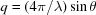is the modulus of the scattering vector, λ is the wavelength and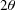is the scattering angle. When relevant, the data were transformed to an absolute scale (coherent differential cross section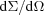) by calculating the normalized scattering intensity from direct beam measurements. The respective scattering-length densities () of CoFe2O4 nanoparticles, laponite platelets, water and sodium citrate are 6.2, 4.1, −0.53 and 2.0 × 1010 cm−2. Here, the scattering of neutrons by magnetic ferrite nanoparticles is mainly due to nuclear interactions with all the nuclei of the sample (Gazeau et al., 2003).

#### 2.6. Small-angle X-ray scattering (SAXS)

The SAXS experiment was done on beamline D11A-SAXS at the Laboratorio Nacional de Luz Sincrotron (LNLS) in Campinas, Brazil (energy range 6–12 keV). All data were collected at a wavelength of 1.756 Å with the sample-to-image plate detector distance of 1.5 m. The samples were placed in 1 mm quartz capillaries. Data treatment was performed using the software FIT2D (ESRF) with the usual corrections included in its routine. The output of this software provides the corrected intensities ready for subtraction of the scattering from the citrated solution and the quartz capillary.

### 3. Small-angle scattering (SAS) analysis

The scattering intensity of a diluted dispersion can be written as a function of q,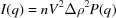, where n is the number of scattering objects of average volume V per volume unit (per cm3) of solution,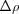is the contrast between the particles and the solvent and P(q) is the particle form factor proportional to the square of the modulus of the particle structure factor [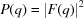]. At sufficiently low q, the scattering may be described by the Guinier law (Guinier & Fournet, 1955) which allows the gyration radius of the scattering object Rg to be determined by writing the low-q expansion of the scattering intensity as I(q) = I(0)exp(-q2 R2g/3). At high values of q, the scattering curve reflects the characteristics of the interface between the scattering object and the solvent (Porod region). In the case of platelets (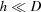, where h is the platelet thickness and D is the platelet diameter, see Fig. 1), the measured scattering intensity scales with q-2 as a characteristic feature for scattering of randomly oriented discs (Ramsay et al., 1990). In the case of spheroidal particles, the scattering intensity in the Porod regime scales with q-4.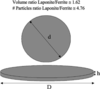Figure 1 Typical dimensions for a clay platelet (h = 1 nm, D = 25 nm) and a magnetic particle (d = 14.0 nm), as well as their volume ratio and concentration ratio (platelets/particles) in the composite material.

Magnetic nanoparticles of ferrofluids are always polydisperse, and the mathematical function which best describes the size distribution is a lognormal law of particle radii: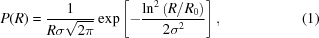where R0 is the characteristic radius of the distribution given by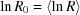andis the standard deviation or polydispersity index. The nth moment of the lognormal distribution is defined by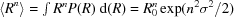. In such a context, we can use a global scattering function proposed by Beaucage (1995) to write the scattering intensity in the case of a lognormal distribution of spherical particles as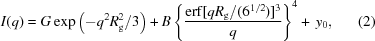where erf(x) is the error function, G is the Guinier pre-factor proportional to the concentration of scattering objects and to the square of the volume, B is a constant pre-factor specific to the kind of power law obtained for high q values and y0 is a term introduced in order to take into account the incoherent scattering. In the case of a lognormal distribution, the parameters Rg and B are related to the characteristic radius and the polydispersity index by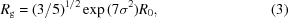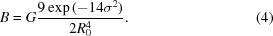The parameters R0,, G and y0 are deduced by fitting the small-angle scattering curves to equation (2)through a nonlinear least-squares fitting procedure with a statistical weighting.

### 4. Results and discussion

Fig. 2displays the powder diffractogram obtained for our synthesized cobalt ferrite magnetic nanoparticles. The positions and relative intensities of the diffracted lines are characteristic of the spinel crystal structure. The average radius is determined according to the Scherrer equation using the half-width of the strongest diffraction peak, and is equal to 7.0 nm. The inset of Fig. 2presents a typical TEM picture of the same sample and it can be seen that the nanoparticles are approximately spherical and polydisperse. Counting about 500 individual particles resulted in a size histogram that is fitted by a lognormal law of parameters R0 = 5.5 nm and= 0.3.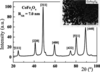Figure 2 X-ray powder diffractogram of the synthesized cobalt ferrite nanoparticles. The inset displays a TEM picture of the same sample.

Fig. 3presents the log–log plot of SANS and SAXS intensities of our pure dilute citrate ferrofluid at a concentration of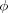= 1 wt%, as a function of q. The measurements collapse onto the same master curve using a scaling factor in between the SAXS and SANS data sets. In both cases we have observed (not shown here) that the scattering intensity is proportional to the volume fraction of magnetic nanoparticles, suggesting a non-interacting regime. For smaller values of q, typically q < 0.01 Å−1, the scattering shows a plateau, also indicating the absence of aggregates and interactions between scattering objects. For larger q values, the scattering intensity scales well with q-4, indicating as expected that the scattering objects are spheroidal ones. The full line in Fig. 3represents the best fit obtained using equation (2)and the deduced values of the lognormal size distribution parameters are R0 = 5.52 nm and= 0.326.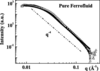Figure 3 Observed SANS (triangles) and SAXS (squares) scattering from pure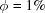citrated cobalt ferrite ferrofluid. The dashed line is a guide line indicating the slope -4. The full line is the best fit to the SAXS data using equation (2). In this case, the measured SAXS data have been multiplied by a factor of 35000 in order to match the SANS data. The vertical axis scale is thus in arbitrary units for SAXS, whereas it is in absolute units of cm−1 for SANS.

Fig. 4shows SAXS scattering from the 0.1 wt% laponite dispersion used in the composite with an ionic strength (I) of 1.5 × 10−3 mol l−1. In such conditions the clay dispersion is an isotropic liquid phase. The full line in Fig. 4shows that the scattering intensity from our laponite dispersion is close to a q-2 behavior as expected for a solution of randomly oriented platelets. Our SAXS scattering at this concentration of 0.1 wt% for laponite is very weak, and very sensitive to possible artifact scattering from the beamstop, from capillary walls etc., which could be responsible for the peak-like behavior observed at low q. We choose not to focus on this possible artifact, but rather observe that the SAXS scattering power of laponite at these concentrations is weak and that a slope of −2 is in agreement with previous observations (Lemaire et al., 2002).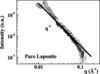Figure 4 Observed SAXS scattering from the pure dilute laponite solution, 0.1 wt%. The full line is a guide line indicating q-2 behavior. Note that in this plot, the vertical scale is as measured and integrated 360° from the isotropic two-dimensional detector data, thus these data (unlike Figs. 3, 5and 6) have not been multiplied with any factor in order to translate vertically.

The laponite–ferrofluid composite sample presents phase separation under a vertically directed applied field of approximately 1.2 T using a discoid rare-earth permanent magnet. The composite separates into a diluted phase of magnetic nanoparticles (upper phase) and a more concentrated one (lower phase). When the field is removed, the sample again becomes just a one-phase liquid. Visually this phase separation is very different from the one observed for the pure ferrofluid, where there is no such phase separation. These observations thus give evidence that these two phases are due only to laponite–ferrofluid particle interactions. Figs. 5and 6present the log–log plots of the scattering intensities of the diluted and concentrated phases of the composite sample. The scattering intensity from pure laponite platelets at our concentration (0.1 wt%) is smaller compared to the scattering obtained from magnetic nanoparticles. This can be seen by comparing Figs. 3and 4. In order to directly compare the scattering power at our laponite concentration of 0.1 wt% with our ferrofluid at= 0.025 wt%, the data in Fig. 3must be multiplied by a factor 0.025/35 000 (Fig. 3is at= 1 wt%, whereas our sample is at= 0.025 wt%, and 35 000 is the scaling factor introduced in order to match the SAXS and SANS data). At q = 0.01 Å−1 this gives about one order of magnitude lower SAXS scattering from laponite as compared to pure ferrofluid for our concentrations. (In SANS we could not observe scattering from 0.1 wt% at all.) The slope of the high-q region follows a q-4 law as expected in the Porod region for non-interacting spheroidal particles. These results thus indicate that the scattering from our laponite–ferrofluid composite is mainly due to the magnetic nanoparticles, both for SAXS and for SANS. Thus we use equation (2)to determine the parameters G, R0,and y0. The resultant fitted parameters are collected in Table 1for the diluted and more concentrated phases. The fitted values for R0 andin both cases agree well with those determined for the diluted pure ferrofluid, showing that for our composite sample the scattering object is the same for both phases. The inset of Figs. 5and 6display the low-q region and the full line is the linear fitting with the Guinier law giving an Rg value [related to R0 by equation (3)] in good agreement with R0 obtained from the fit with the global scattering function. The R0 andvalues obtained compare well with the RXR value using the following empirical relationship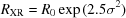(Tronc & Bonnin, 1985). The ratio of the Guinier pre-factor determined from these SAS curve analyses gives the ratio between the concentrations of the phases. The SANS data have been scaled to fit with the SAXS data in the middle-q region, and we find relatively more incoherent scattering (at high q) for neutrons compared to X-rays. The incoherent scattering observed in SANS is mostly due to incoherent scattering from water.

 Table 1Fitted parameters for the two phases of the composite
Fitted parameters Diluted phase Concentrated phase
G 6.30 ± 1.15 9.6 ± 1.1
R0 (nm) 5.79 ± 0.20 5.86 ± 0.300.32 ± 0.06 0.33 ± 0.06
y0 0.0012 0.0000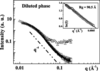Figure 5 Observed SANS (triangles) and SAXS (squares) scattering curves from the dilute phase of the composite sample. The dashed line is a guide line indicating the slope -4, and the full line is the best fit using equation (2). The inset displays the low-q region where the full line is a fit to the Guinier law. In this case, the measured SAXS data have been multiplied by a scale factor in order to match the SANS data. The vertical axis scale is thus in arbitrary units for SAXS, whereas it is in absolute units of cm−1 for SANS.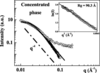Figure 6 Observed SANS (triangles) and SAXS (squares) scattering from the concentrated phase of the composite sample. The dashed line is a guide line indicating the slope -4 and the full line is the best fit using equation (2). The inset displays the low-q region where the full line is a fit to the Guinier law. In this case, the measured SAXS data have been multiplied by a scale factor in order to match the SANS data. As in Fig. 5, the vertical axis scale is in arbitrary units for SAXS, whereas it is in absolute units of cm−1 for SANS.

### 5. Conclusions

A composite material was obtained from the mixture of an isotropic phase laponite solution with a diluted citrate ferrofluid for a given magnetic nanoparticle volume fraction. Such a laponite–ferrofluid composite material raises general questions concerning colloidal stability. During sample mixing, the pH and ionic strength parameters of the final product were optimized in order to respect the interval of stability of each component. Small-angle scattering experiments were performed in order to investigate the nanostructure of the composite sample. The scattering is much more intense for the magnetic nanoparticles than for the laponite platelets, which implies that the composite scattering signal is mainly due to the magnetic nanoparticles. The scattering obtained by SANS and SAXS collapse onto a common master curve, but differs at high q where more incoherent scattering is observed in SANS. In both cases, SAXS and SANS, the scattering intensity is proportional to the volume fraction of magnetic particles present in each phase, and this indicates for both phases a non-interacting particle system from the scattering point of view in the present q range. Fitting the scattering intensities to a global scattering function including the size polydispersity allows us to deduce the characteristic radius and polydispersity index of the scattering object present in the composite sample, and to show that it is the same as for the pure ferrofluid sample. Our microscopy observations clearly show that our magnetic particles interact with the laponite particles, i.e. laponite-mediated interactions in between magnetic particles. Future work should include investigating various other concentrations of laponite and magnetic particles in addition to the ones reported here.

### Acknowledgements

The authors wish to acknowledge the help from the staff at beamline D11A-SAXS at the Laboratorio Nacional de Luz Sincrotron (LNLS) during the SAXS experiments. This work was supported by the Brazilian agencies CAPES and CNPq, and by the Research Council of Norway (RCN) through the NANOMAT, FRINAT and SUP Programs: RCN project numbers 152426/431, 154059/420 and 148865/432, as well as 138368/V30 and SUP154059/420.

### ReferencesJOURNAL OFAPPLIEDCRYSTALLOGRAPHY